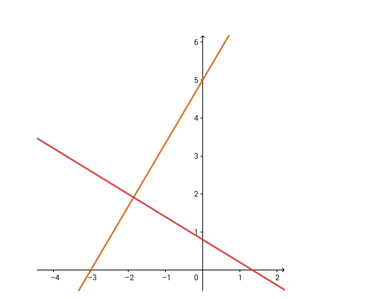Deepak Scored 45->99%ile with Bounce Back Crack Course. You can do it too!

# Find the equation of the line that has x - intercept - 3

Question:

Find the equation of the line that has x - intercept - 3 and which is perpendicular to the line 3x + 5y = 4

Solution:Given: The given line is $3 x+5 y=4$. The perpendicular line has an $x-$ intercept of $-3$.

Formula to be used: The product of slopes of two perpendicular lines $=-1$.

The slope of this line is $-3 / 5$.

$\therefore$ the slope of the perpendicular line $=$

$\frac{-1}{-3 / 5}=5 / 3$

The equation of the line can be written in the form

$y=\left(\frac{5}{3}\right) x+c$

(c is the y - intercept)

This line intercepts the x - axis when y = 0.

So, the x - intercept:

$0=\left(\frac{5}{3}\right) \mathrm{x}+\mathrm{c}$ i.e. $\mathrm{x}=-\frac{3 \mathrm{c}}{5}$

Now, it is given that the x - intercept is - 3

$\therefore-\frac{3 c}{5}=-3$ i.e. $c=5$

The required equation of the line is

$y=\left(\frac{5}{3}\right) x+5$

i.e. $5 x-3 y+15=0$